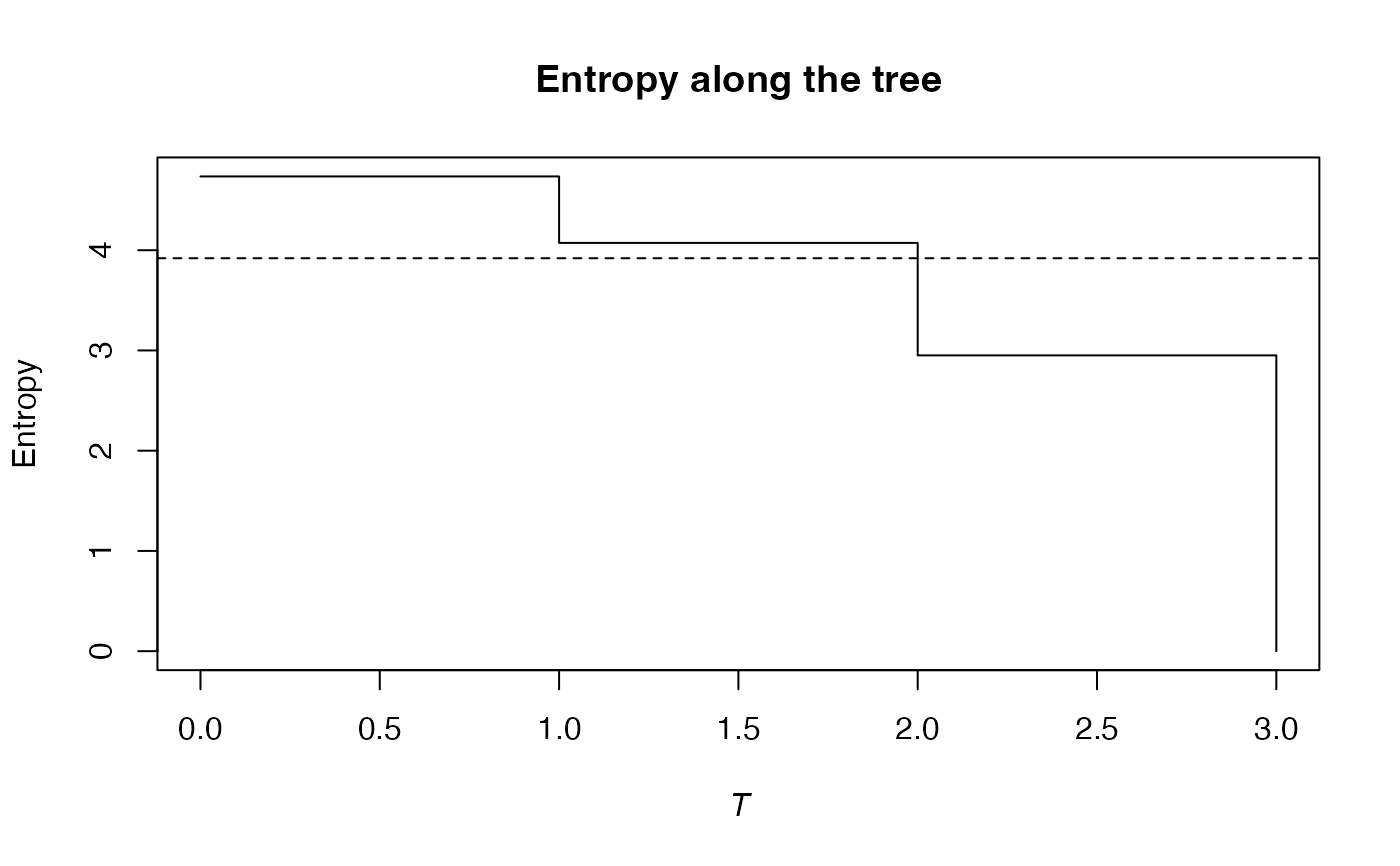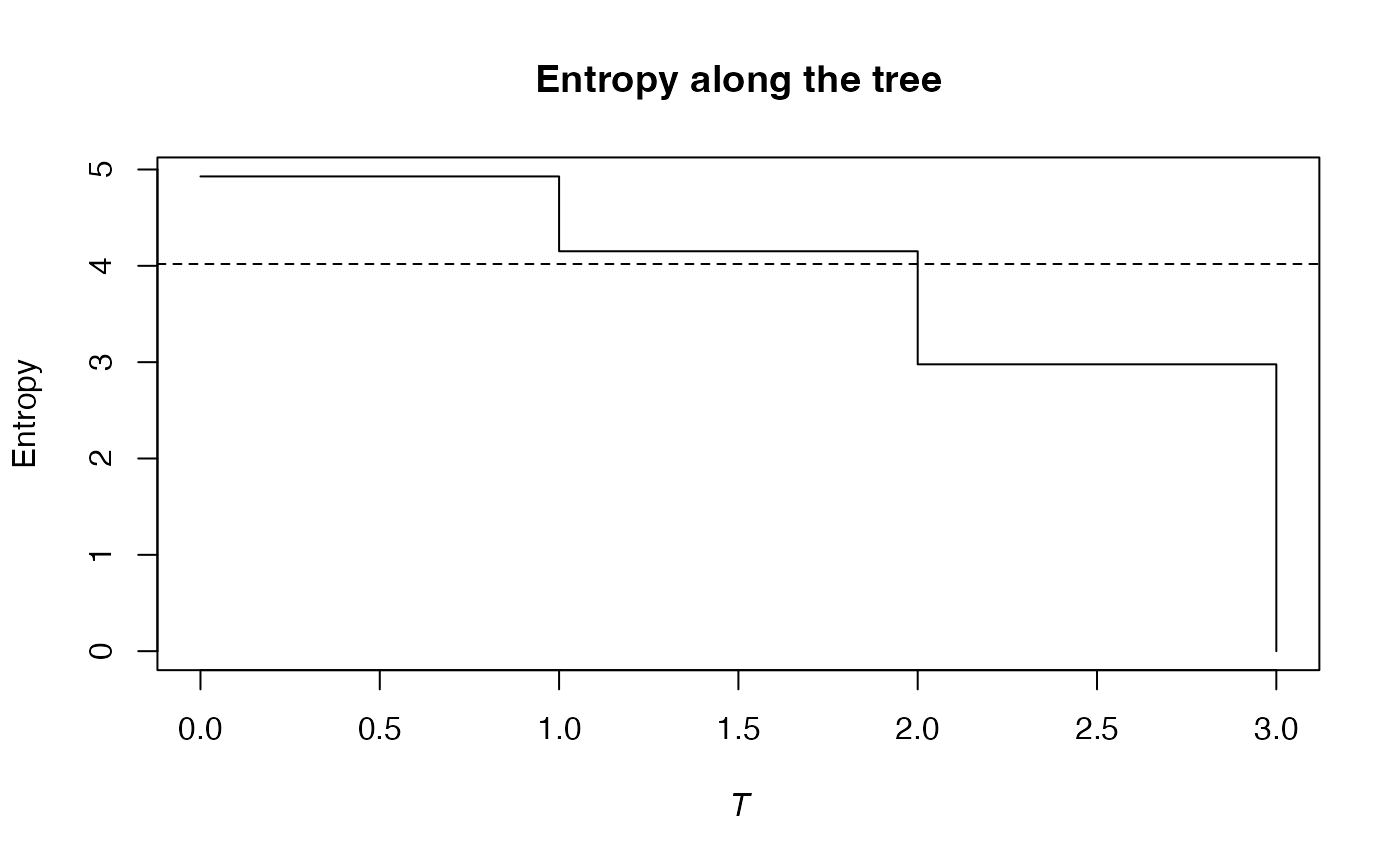Calculates the phylogenetic entropy of order $$q$$ of a probability vector.

PhyloEntropy(NorP, q = 1, Tree, Normalize = TRUE, ...)
bcPhyloEntropy(Ns, q = 1, Tree, Normalize = TRUE, Correction = "Best",
SampleCoverage = NULL, CheckArguments = TRUE)
# S3 method for ProbaVector
PhyloEntropy(NorP, q = 1, Tree, Normalize = TRUE,
..., CheckArguments = TRUE, Ps = NULL)
# S3 method for AbdVector
PhyloEntropy(NorP, q = 1, Tree, Normalize = TRUE, Correction = "Best",
..., CheckArguments = TRUE, Ns = NULL)
# S3 method for integer
PhyloEntropy(NorP, q = 1, Tree, Normalize = TRUE, Correction = "Best",
..., CheckArguments = TRUE, Ns = NULL)
# S3 method for numeric
PhyloEntropy(NorP, q = 1, Tree, Normalize = TRUE, Correction = "Best",
..., CheckArguments = TRUE, Ps = NULL, Ns = NULL)
is.PhyloEntropy(x)
# S3 method for PhyloEntropy
summary(object, ...)

## Arguments

Ps

A probability vector, summing to 1.

Ns

A numeric vector containing species abundances.

NorP

A numeric vector, an integer vector, an abundance vector (AbdVector) or a probability vector (ProbaVector). Contains either abundances or probabilities.

q

A number: the order of entropy. Default is 1.

Tree

An object of class hclust, phylo, phylog or PPtree. The tree must be ultrametric.

Normalize

If TRUE (default), the Total entropy returned by the function is normalized by the height of the tree (it is the weighted average value of the entropy in each slice).
If FALSE, it is the unnormalized weighted sum of the results.

Correction

A string containing one of the possible corrections supported by Tsallis.

SampleCoverage

The sample coverage of Ns calculated elsewhere. Used to calculate the gamma diversity of meta-communities, see details.

CheckArguments

Logical; if TRUE, the function arguments are verified. Should be set to FALSE to save time when the arguments have been checked elsewhere.

x

An object to be tested or plotted

object

A PhyloEntropy object to be summarized.

...

Additional arguments to be passed to the generic methods.

## Details

The phylogenetic entropy is its generalization of HCDT entropy to unequal species distances (Pavoine et al., 2009).

Calculation relies on Tsallis and PhyloApply.

Intervals separate two cuts in a tree: no node is found at heights contained in an interval.

Bias correction requires the number of individuals to estimate sample Coverage. Use bcPhyloEntropy and choose the Correction.

The functions are designed to be used as simply as possible. PhyloEntropy is a generic method. If its first argument is an abundance vector, an integer vector or a numeric vector which does not sum to 1, the bias corrected function bcPhyloEntropy is called. Explicit calls to bcPhyloEntropy (with bias correction) or to PhyloEntropy.ProbaVector (without correction) are possible to avoid ambiguity. The .integer and .numeric methods accept Ps or Ns arguments instead of NorP for backward compatibility.

The size of a metacommunity (see MetaCommunity) is unknown so it has to be set according to a rule which does not ensure that its abundances are integer values. Then, classical bias-correction methods do not apply. Providing the SampleCoverage argument allows applying the "ChaoShen" and "Grassberger" corrections to estimate quite well the entropy. DivPart and GammaEntropy functions use this tweak.

## Note

The tree must contain all species of the probability vector. If it contains extra species, computation time will just be increased.

## Value

An object of class PhyloEntropy is a list:

Distribution

The distribution used to calculate entropy

Function

The function used to calculate entropy

Tree

The functional or phylogenetic tree used to calculate entropy

Normalized

Logical. Indicates whether phyloentropy is normalized or proportional to the height of the tree.

Type

The type of entropy ("alpha", "beta" or "gamma").

Order

The order of entropy $$q$$.

Cuts

A named vector containing values of neutral entropy along the tree. Names are cut ends, i.e. the ends of intervals (the first interval starts at 0 for leaves, the max value is the height of the tree).

Total

A value equal the total entropy multiplied by the tree height if Normalize is FALSE.

is.PhyloEntropy returns TRUE if the object is of class PhyloEntropy. summary.PhyloEntropy returns a summary of the object's value. PhyloEntropy objects can be plotted by plot.PhyloValue because PhyloEntropy objects are also of class PhyloValue.

Tsallis, PhyloDiversity

## Examples

# Load Paracou data (number of trees per species in two 1-ha plot of a tropical forest
#      and their taxonomy)
data(Paracou618)
# Ps is the vector of probabilities
Ps <- as.ProbaVector(Paracou618.MC$Ps) # Calculate the phylogenetic Shannon entropy of the plot summary(PhyloEntropy(Ps, 1, Paracou618.Taxonomy) -> e) #> alpha or gamma phylogenetic or functional entropy of order 1 of distribution - #> with correction: None #> Phylogenetic or functional entropy was calculated according to the tree #> Paracou618.Taxonomy #> #> Entropy is normalized #> #> Entropy equals: 3.919941 plot(e)# Ns is the vector of abundances of the metacommunity Ns <- as.AbdVector(Paracou618.MC$Ns)
# Calculate the phylogenetic Shannon entropy of the plot
summary(bcPhyloEntropy(Ns, 1, Paracou618.Taxonomy, Correction = "Best") -> e)
#> alpha or gamma phylogenetic or functional entropy of order 1 of distribution -
#>  with correction: Best
#> Phylogenetic or functional entropy was calculated according to the tree
#> Paracou618.Taxonomy
#>
#> Entropy is normalized
#>
#> Entropy equals: 4.018993
plot(e)